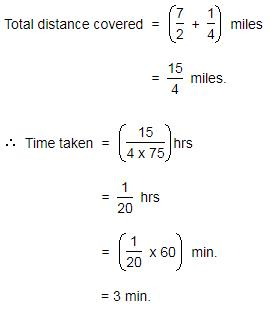Courses

# Test: Problems On Trains- 1

## 10 Questions MCQ Test Logical Reasoning (LR) and Data Interpretation (DI) | Test: Problems On Trains- 1

Description
This mock test of Test: Problems On Trains- 1 for LR helps you for every LR entrance exam. This contains 10 Multiple Choice Questions for LR Test: Problems On Trains- 1 (mcq) to study with solutions a complete question bank. The solved questions answers in this Test: Problems On Trains- 1 quiz give you a good mix of easy questions and tough questions. LR students definitely take this Test: Problems On Trains- 1 exercise for a better result in the exam. You can find other Test: Problems On Trains- 1 extra questions, long questions & short questions for LR on EduRev as well by searching above.
QUESTION: 1

### A train moves past a post and a platform 264 m long in 8 seconds and 20 seconds respectively. What is the speed of the train?

Solution: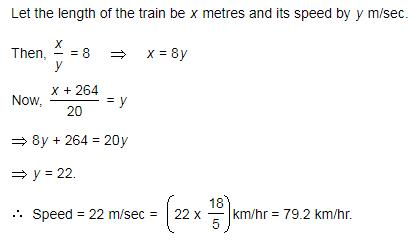QUESTION: 2

### A train overtakes two persons who are walking in the same direction to that of the train at 2 kmph and 4 kmph and passes them completely in 9 and 10 seconds respectively. What is the length of the train?

Solution: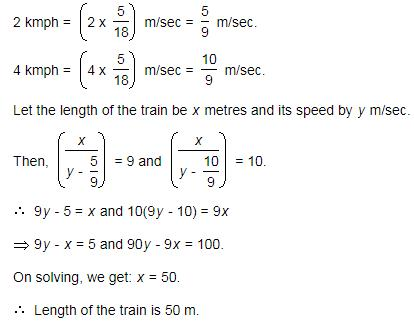QUESTION: 3

### A train runs at the speed of 72 kmph and crosses a 250 m long platform in 26 seconds. What is the length of the train?

Solution: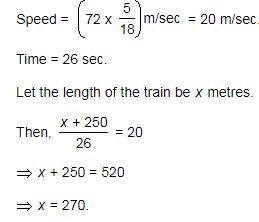QUESTION: 4

A train is traveling at 48 kmph . It crosses another train having half of its length , traveling in opposite direction at 42 kmph, in 12 seconds. It also passes a railway platform in 45 seconds. What is the length of the platform?

Solution:

Let the length of the train traveling at 48 kmph be 2x meters.

And length of the platform is y meters.

Relative speed of train = (48+42) kmph

= (90*5/18) = 25 m/sec;

And 48 kmph = 48*5/18 = 40/3 m/sec.

According to the question,

(2x +x)/25 = 12;

Or, 3x = 12*25 = 300;

Or, x = 300/3 = 100m

Then, length of the train = 2x = 100*2 = 200m.

200+y/(40/3) = 45;

600+3y = 40*45;

Or, 3y = 1800-600 = 1200;

Or, y = 1200/3 = 400 m.

Length of the platform = 400 m.

QUESTION: 5

Two trains, one from P to Q and the other from Q to P, start simultaneously. After they meet, the trains reach their destinations after 9 hours and 16 hours respectively. The ratio of their speeds is

Solution: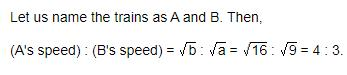QUESTION: 6

Two trains having equal lengths, take 10 seconds and 15 seconds respectively to cross a post. If the length of each train is 120 meters, in what time (in seconds) will they cross each other when traveling in opposite direction?

Solution: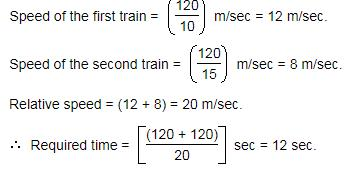QUESTION: 7

Two trains, each 100 m long are moving in opposite directions. They cross each other in 8 seconds. If one is moving twice as fast the other, the speed of the faster train is

Solution: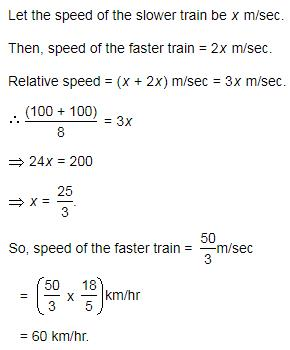QUESTION: 8

A 270 metres long train running at the speed of 120 kmph crosses another train running in opposite direction at the speed of 80 kmph in 9 seconds. What is the length of the other train?

Solution: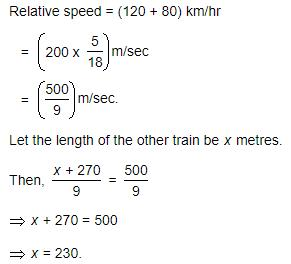QUESTION: 9

A train passes a platform in 36 seconds. The same train passes a man standing on the platform in 20 seconds. If the speed of the train is 54 km/hr, The length of the platform is

Solution: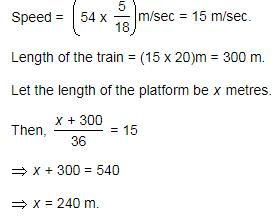QUESTION: 10

A train having a length of 1/4 mile , is traveling at a speed of 75 mph. It enters a tunnel 3 ½ miles long. How long does it take the train to pass through the tunnel from the moment the front enters to the moment the rear emerges?

Solution: